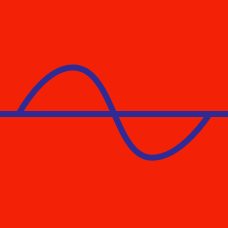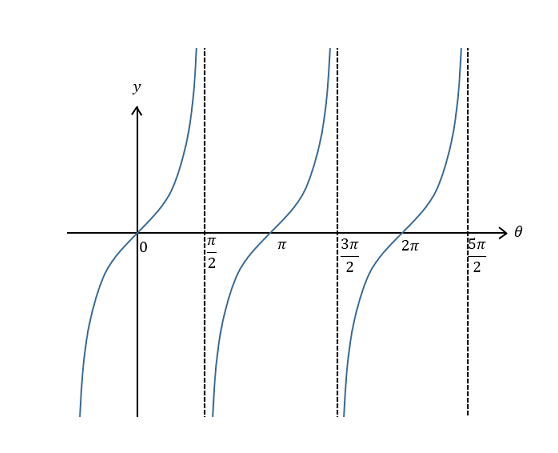Geometry

# Tangent and Cotangent Graphs

What is the value of $\theta$ satisfying $8\tan^2\theta = 24$ and $-\frac{\pi}{2} < \theta < \frac{\pi}{2}?$

What is the minimum value of the function $y= \tan\theta+18,$ where $\frac{\pi}{3} \leq \theta < \frac{\pi}{2} ?$

What is the range of $\theta$ that satisfies $\tan\theta +6 \geq \cot\theta +6$ and $0 \leq \theta < \pi ?$

What is the period of the function $y=\cot\frac{\theta}{2} ?$Which of the following functions corresponds to the above graph?

×